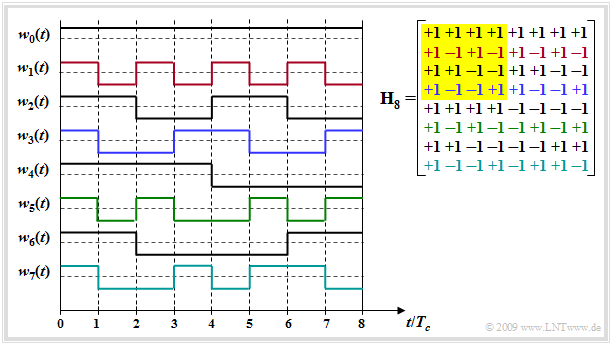# Generation of Walsh functions

## Program description

This applet allows to display the Hadamard matrices  $\mathbf{H}_J$  for the construction of the Walsh functions  $w_j$.  The factor  $J$  of the band spreading as well as the selection of the individual Walsh functions  (by means of a blue border around rows of the matrix)  can be changed.

## Theoretical background

### Application

The  Walsh functions  are a group of periodic orthogonal functions.  Their application in digital signal processing mainly lies in the use for band spreading in CDMA systems, for example the mobile radio standard UMTS.

• Due to their orthogonal properties and the favourable periodic cross-correlation function  $\rm (PCCF)$, the Walsh functions represent optimal spreading sequences for a distortion-free channel and a synchronous CDMA system.  If you take any two lines and form the correlation (averaging over the products), the PCCF value is always zero.
• In asynchronous operation  (example:   uplink of a mobile radio system)  or de-orthogonalization due to multipath propagation, Walsh functions alone are not necessarily suitable for band spreading.
• In terms of  $\rm (PACF)$  (periodic autocorrelation function) these sequences are not as good:  Each individual Walsh function has a different PACF and each individual PACF is less good than a comparable pseudo noise  $\rm (PN)$  sequence. That means:   The synchronization is more difficult with Walsh functions than with PN sequences.

### Construction

The construction of Walsh functions can be done recursively using the  Hadamard matrices.

• A Hadamard matrix  $\mathbf{H}_J$  of order  $J$  is a  $J\times J$  matrix, which contains line by line the  $\pm 1$  weights of the Walsh sequences.
• The orders of the Hadamard matrices are fixed to powers of two, i.e.  $J = 2^G$  applies to a natural number  $G$. Starting from $\mathbf{H}_1 = [+1]$ and
$$\mathbf{H}_2 = \left[ \begin{array}{rr} +1 & +1\\ +1 & -1 \\ \end{array}\right]$$

the following relationship applies to the generation of further Hadamard matrices:

$$\mathbf{H}_{2N} = \left[ \begin{array}{rr} +\mathbf{H}_N & +\mathbf{H}_N\\ +\mathbf{H}_N & -\mathbf{H}_N \\ \end{array}\right]$$

$\text{Example:}$  The graphic shows the Hadamard matrix  $\mathbf H_8$  (right) and the  $J\hspace{-0.09cm} -\hspace{-0.09cm}1$  spreading sequences which can be constructed with it.Walsh spreading sequences  $(J = 8)$  and Hadamard matrix  $\mathbf H_8$
• Only  $J\hspace{-0.09cm} -\hspace{-0.09cm}1$, because the unspreaded sequence  $w_0(t)$  is usually not used.
• Please note the color assignment between the lines of the Hadamard matrix and the spreading sequences  $w_j(t)$.
• The submatrix  $\mathbf H_4$  is highlighted in yellow.

## How to use the applet

(A)     Selection of  $G$   ⇒   Band spread factor:  $J= 2^G$

(B)     Selection of the Walsh function  $w_j$  to be marked

## About the authors

This interactive calculation tool was designed and realized at the  Lehrstuhl für Nachrichtentechnik  $\rm (LNT)$  of the  Technical University of Munich  $\rm (TUM)$.

• The first German version was created in 2007 by  Thomas Großer   in the context of his diploma thesis with "FlashMX–Actionscript"  (Supervisor:  Günter Söder).
• 2018/2019 the applet was converted on "HTML5" and redesigned by  Carolin Mirschina  (Engineering practice, supervisor:  Tasnád Kernetzky ).
• 2020 this English version was made by  Carolin Mirschina  (working student) and  Günter Söder.  Translation using "www.DeepL.com/Translator" (free version).